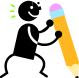## Monday, May 17, 2010

### Guess The Number

Introduce younger students to logic problems using Mathwire's Guess the Number collection.  Give students more practice in using the hundred board to solve number clue problems. Once again, students solve the problem clue by clue. With this set, however, students must use multiplication, money, and measurement facts to correctly solve the problem and guess the number.

Here's a sample problem:
• The number is greater than the number of pennies in a quarter.
• The number is less than the number of pennies in five dimes.
• The number is an odd number.
• If you count by 5s, you say the number.
• The sum of the digits is 8.
What is the number?

Check out Guess the Number on Mathwire to download more problems and their solutions.  Be sure to read suggestions for using these problems in math classes.  After solving several, encourage students to write their own original Guess the Number logic problems.

## Saturday, May 15, 2010

### Hundred Board Logic ProblemsThese original Mathwire Hundred Board Logic problems are designed to help students use a hundred board to solve problems.  Students read each clue, then eliminate numbers on the hundred board that do not fit the clue.  After reading through all clues, students should be left with only one solution.  They should check their solution by rereading each clue to be sure their solution fits.  These problems were field-tested in Grades 3-8 classrooms and students loved the experience because the step-by-step process enabled them to be successful at solving these problems.

Enrichment:  After students have solved several of these problems, they may be challenged to create their own original problems.  Provide blank 100 board and logic problem templates.  Students should start by circling their target solution.  They then write clues that will eliminate all other numbers.  Finally, students should exchange problems and use peer review to edit their problems, clarifying and modifying clues to eliminate any confusion.Creating original problems is a higher-order thinking skill (Synthesis on Bloom's Taxonomy) that extends students' problem solving ability and requires flexible thinking about mathematical concepts, skills and vocabulary terms.  Student-created problems may be added to the classroom math center and used on game days as a problem-solving center.  Additionally, these problems may be used in the initial lesson in subsequent years.  Consider publishing the problems with the author's name and copyright year.  Students love seeing their names in print!

Materials:
• Read more about Hundred Board Logic Problems including classroom management suggestions and ideas for a cooperative learning lesson.  The discussion includes PDF documents for all problems and templates needed for this lesson.

## Wednesday, May 12, 2010

### Tribulation Game

This game challenges students to find equivalent names for numbers.   In Tribuation, students must search the gameboard for 3 numbers in a row (vertically, horizontally or diagonally as in a word search) that combine to make the target number.   In this game, however, there is a prescribed formula for combining the numbers.   Students must multiply the first two numbers then add or subtract the third number to produce the target number

Students quickly learn that any string of three numbers produce four different results since the string of numbers can be considered both forwards and backwards as in word searches.   Consider that the numbers 3, 5 and 8 are in a horizontal row.   These three numbers produce the following results when combined in the formula:
• 3 x 5 + 8 = 23
• 3 x 5 - 8 = 7
• 8 x 5 + 3 = 43
• 8 x 5 - 3 = 37
Note that the student may not begin with the middle number.   Once again, students will perform many mental calculations in a search for a viable solution on the gameboard.

This game was originally a board game in the 1980s but has been developed into a classroom game using a gameboard template designed to be inserted in a sheet protector and used with dry-erase pens so that students can easily circle and record solutions.   Two different approaches are effective in using this game:   (1) students search for one solution for 6-8 different target numbers, and (2) students search for many different solutions for one number.   Samples of both types of activities are included in the Tribulation directions packet for teachers.

Read more about the Tribulation Game on Mathwire.com to download instructions and templates that can be inserted in sheet protectors for daily use.